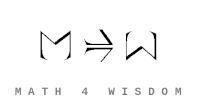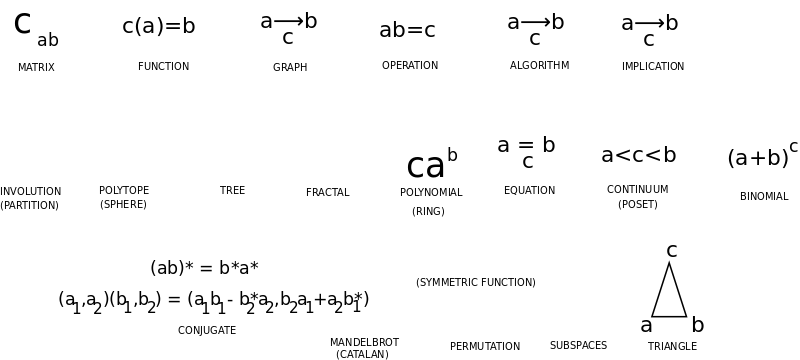文章 发现 ms@ms.lt +370 607 27 665 My work is in the Public Domain for all to share freely. 读物 书 影片 维基百科 Software Upload See: Math Big Picture 数学概念 Overview all of the concepts in math and how they arise. What is mathematical knowledge? How does it arise, develop and take the form of objects, concepts, theorems and endeavors? What are the roles of abstraction-generalization and concrete examples? Define the most basic concepts, especially the categories below. Organize the basic concepts. Show how they arise from the way we relate several variables and interpret duality. Particular concepts Compare a Fourier series and a generating function. How is a single basis element isolated, calculated, in each case? On this page I am organizing a list of mathematical concepts that I wish to be able to account for. Sources of concepts include: Basic ideas The most central concept in Math is duality, which is the basis for logic. The most fundamental symmetry is that of choice, as given by the ways we may interpret the binomial theorem, for it underlies the symmetry of algebraic expansions.Matematikos savokų pagrindas yra dvejybinis-trejybinis - operacijos jungia du narius trečiu nariu. Matricų elementai sieja du narius ir išgauna trečią. Kategorijų teorija panašiai. O geometrija lygiaverčiai sieja tris narius trikampiais, įvairiai suprastais. Tad tai paaiškintų geometrijos svarbą. Mathematical concepts Objects? and their Symmetry combinatorial principles mathematical shapes polygons, polyhedra and polytopes regular polytopes - points on a circle or sphere, etc. partition topics factorial and binomial topics convexity topics Binomial, Choice, Probability, Entropy Definite / deterministic (the) vs. Indefinite / Nondeterministic (a) - P vs. NP Axiom of Choice Numbers Real, Complex, Quaternion Bott periodicity - Clifford Algebra shift clock - and Octonions Octonions Trialities and spinors Magic Square of Lie algebras matrices continuum order topics numeral system topics properties of sets of reals exceptional set concepts fractal topics Functions mathematical functions special functions and eponyms transforms Fourier-related transforms polynomial topics exponential topics logarithm topics Equations equations inequalities mathematical identities formulae involving pi Subspaces curves mathematical knots and links surfaces algebraic surfaces manifolds examples in general topology Operations small groups finite simple groups simple Lie groups Table of Lie groups complex reflection groups permutation topics Algorithms algorithms complexity classes Proofs axioms conjectures lemmas mathematical proofs theorems Particular concepts Catalan numbers are related to semantics and to the generating function of the mandelbtot set Universal cover. Wikipedia: The concept of a universal cover was first developed to define a natural domain for the analytic continuation of an analytic function. The idea of finding the maximal analytic continuation of a function in turn led to the development of the idea of Riemann surfaces. The power series defined below is generalized by the idea of a germ. The general theory of analytic continuation and its generalizations are known as sheaf theory. Covering spaces. Wikipedia: Covering spaces play an important role in homotopy theory, harmonic analysis, Riemannian geometry and differential topology. In Riemannian geometry for example, ramification is a generalization of the notion of covering maps. Covering spaces are also deeply intertwined with the study of homotopy groups and, in particular, the fundamental group. An important application comes from the result that, if X is a "sufficiently good" topological space, there is a bijection between the collection of all isomorphism classes of connected coverings of X and the conjugacy classes of subgroups of the fundamental group of X. Characteristic class? of different kinds are related to the classical linear groups. Notes on concepts Function can be partial, whereas a permutation maps completely. Intrinsic ambiguity of propositions - every proposition is a general rule, which can be questioned or applied. Consider how concepts arise. Rationality and irrationality relate linearity and integrality by way of a linear equation which may or may not have a solution, and solutions which may or may not have such an equation. Challenge problems: Determine whether {$\pi + e$} is rational or irrational. Determine whether {$\pi^e$} is rational or irrational. Basic concept: Orientation (of a simplex). Relates to determinant, homology, etc. Vector spaces are basic. What is basic about scalars? They make possible proportionality. Basic concepts are the ways of figuring things out. Basic concept - orientation = parity. Randomness. Random phenomena organize themselves around a critical boundary. Real line models separation (by cutting) and connectedness (by continuity). The separating cuts become locations (points) in their own right. The definition of a completely new and distinct entity comes from the oneness in the fluttering that dances in and out of all of the existing entitities. That fluttering cannot be any one of them. Fourier analysis. The signal = the total stream = onesome.
Šis puslapis paskutinį kartą keistas September 18, 2020, at 08:39 PM